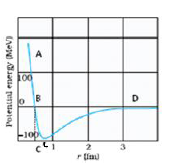Draw a plot of potential  energy of a pair of nucleons as a function of their separations. Mark the regions where the nuclear force (i) attractive and (ii)repulsive. Write any two characteristic features of nuclear forces.(1) marks

For r<OB, repulsive                                                             (1/2) marks

For r>OB force is attractive                                                  (1/2) marks

Nuclear forces are

i) very strong

ii) charge dependent                                                           (1/2) marks

iii) show saturation                                                              (1/2) marks

iv) spin dependent

(any two)

Difficulty Level: